# Causal inference and potential outcome framework

In this blog, we would like to introduce basic concepts in causal inference and the potential outcome framework.

# 1.Causality terminology

1. Unit: The fundamental notion is that causality is tied to action (or manipulation, treatment, or intervention), applied to a unit. A unit here can be a physical object, a firm, a person, at a particular point in time. The same physical object or person at a different time in a different unit. For instance, when you have a headache and you decide to take an aspirin to relieve your headache, you could also have chosen not to take the aspirin, or you could have chosen to take alternative medicine. In this framework, articulating with precision the nature and timing of the action sometimes require a certain amount of imagination. For example, if we define race solely in terms of skin color, the action might be a pill that alters only skin color. Such a pill may not currently exist (but, then, neither did surgical procedures for heart transplants hundreds of years ago), but we can still imagine such action.
2. Active treatment vs. Control treatment: Often, one of these actions corresponds to more active treatment (e.g., taking an aspirin) in contrast to a more passive action (e.g., not taking the aspirin). We refer to the first action as the active treatment, the second action as the control treatment
3. Potential Outcome: given a unit and a set of actions, we associate each action-unit pair with a potential outcome. We refer to these outcomes as potential outcomes because only one will ultimately be realized and therefore possibly observed: the potential outcome corresponding to the taken. The other potential outcomes cannot be observed because the corresponding actions that would lead to them being realized were not taken.
4. Causal Effect: The causal effect of one action or treatment relative to another involves the comparison of these potential outcomes, one realized and the others not realized and therefore not observable.

Suppose we have a ‘treatment’ variable A with two levels: 1 and 0 and an outcome variable Y with two levels: 1 (death) and 0 (survival). Treatment A has a causal effect on an individual’s outcome Y if the potential outcomes under a = 1 and a = 0 are different. The causal effect of the treatment involves the comparison of these potential outcomes. A causes B if: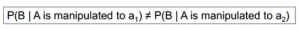For example, consider the case of a single unit, I, at a particular point in time, contemplating whether or not to take an aspirin for my headache. That is, there are two treatment levels, taking an aspirin, and not taking aspirin. There are therefore two potential outcomes, Y(Aspirin) and Y(No Aspirin), one for each level of the treatment.

Table 1: illustrates this situation assuming the values Y(Aspirin) = No Headache, Y (No Aspirin) = Headache.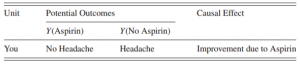1. A fundamental problem of causal inference: There are two important aspects of the definition of a causal effect. First, the definition of the causal effect depends on the potential outcomes, but it does not depend on which outcome is observed. Specifically, whether I take aspirin (and am therefore unable to observe the state of my headache with no aspirin) or do not take aspirin (and am thus unable to observe the outcome with an aspirin) does not affect the definition of the causal effect. Second, the causal effect is the comparison of potential outcomes, for the same unit, at the same moment in time post-treatment. In particular, the causal effect is not defined in terms of comparisons of outcomes at different times, as in a before-and-after comparison of my headache before and after deciding to take or not to take the aspirin. “The fundamental problem of causal inference” (Holland, 1986, p.947) is therefore the problem that at most one of the potential outcomes can be realized and thus observed. If the action you take is Aspirin, you observe Y(Aspirin) and will never know the value of Y(No Aspirin) because you cannot go back in time.
2. Causal Estimands / Average Treatment Effect: For a population of units, indexed by i = 1,…, N. Each unit in this population can be exposed to one of a set of treatments.
• Let Ti (or Wi elsewhere)denote the set of treatments to which unit I can be exposed.

Ti = T = {0, 1}

• For each unit i, and for each treatment in the common set of treatments, there are corresponding potential outcome Yi(0) and Yi(1).
• Comparison of Y1(1) and Yi(0) are unit-level causal effects

Yi(1) – Yi(0)

# 2 .Potential Outcomes Framework

## 2.1       Introduction

The potential outcome framework, formalized for randomized experiments by Neyman (1923) and developed for observational settings by Rubin (1974), defines for all individuals such potential outcomes, only some of which are subsequently observed. This framework dominates applications in epidemiology, medical statistics, and economics, stating the conditions under causal effects can be estimated in rigorous mathematical language

The potential outcomes approach was designed to quantify the magnitude of the causal effect of a factor on an outcome, NOT to determine whether it is a cause or not. Its goal is to estimate the effects of “cause”, not causes of an effect. Quantitative counterfactual inference helps us predict what would happen under different circumstances, but is agnostic in saying which is a cause or not.

## 2.2       Counterfactual

The potential outcome is the value corresponding to the various levels of treatment: Suppose we have a ‘treatment’ variable X with two levels: 1 (treat) and 0 (not treat) and an outcome variable Y with two levels: 1 (death) and 0 (survival). If we expose a subject, we observe Y1 but we do not observe Y0. Indeed, Y0 is the value we would have observed if the subject had been exposed. The unobserved variable is called a counterfactual. The variables (Y0, Y1) are also called potential outcomes. We have enlarged our set of variables from (X, Y) to (X, Y, Y0, Y1). A small dataset might look like this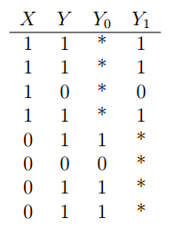The asterisks indicate unobserved variables. Causal questions involve the distribution p(y0, y1) of the potential outcomes. We can interpret p(y1) as p(y|set X = 1) and we can interpret p(y0) as p(y|set X = 0). For each unit, we can observe at most one of the two potential outcomes, the other is missing (counterfactual).

Causal inference under the potential outcome framework is essentially a missing data problem. Suppose now that X is a binary variable that represents some exposure. So X = 1 means the subject was exposed and X = 0 means the subject was not exposed. We can address the problem of predicting Y from X by estimating E(Y|X = x). To address causal questions, we introduce counterfactuals. Let Y1 denote the response if the subject is exposed. Let Y0 denote the response if the subject is not exposed. Then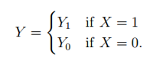Potential outcomes and assignments jointly determine the values of the observed and missing outcomes: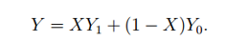Since it is impossible to observe the counterfactual for a given individual or set of individuals. Instead, evaluators must compare outcomes for two otherwise similar sets of beneficiaries who are and are not exposed to the intervention, with the latter group representing the counterfactual

## 2.3       Confounding

In some cases, it is not feasible or ethical to do a randomized experiment and we must use data from observational (non-randomized) studies. Smoking and lung cancer is an example. Can we estimate causal parameters from observational (non-randomized) studies? The answer is: sort of

In an observational study, the treated and untreated groups will not be comparable. Maybe the healthy people chose to take the treatment and the unhealthy people didn’t. In other words, X is not independent. The treatment may have no effect but we would still see a strong association between Y and X. In other words, a (correlation) may be large even though q (causation) = 0.

Here is a simplified example. Suppose X denotes whether someone takes vitamins and Y is some binary health outcome (with Y = 1 meaning “healthy”)In this example, there are only two types of people: healthy and unhealthy. The healthy people have (Y0, Y1) = (1,1). These people are healthy whether or not they take vitamins. The unhealthy people have (Y0, Y1)= (0,0). These people are unhealthy whether or not they take vitamins.

The observed data are: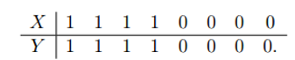In this example, q = 0 but a = 1. The problem is that people who choose to take vitamins are different from people who choose not to take vitamins. That’s just another way of saying that X is not independent of (Y0, Y1).

To account for the differences in the groups, we can measure confounding variables. These are the variables that affect both X and Y. These variables explain why the two groups of people are different. In other words, these variables account for the dependence between X and  Y. By definition, there are no such variables in a randomized experiment. The hope is that if we measure enough confounding variables then, perhaps the treated and untreated groups will be comparable, condition on Z. This means that  is independent of  conditional on Z.

## 2.4       Measuring the Average Causal Effect

The mean treatment effect or mean causal effect is defined by

E(Y1) – E(Y0) = E(Y|set X=1) – E(Y|set X=0)

The parameter q has the following interpretation: q is the mean response if we exposed everyone minus the mean response if we exposed no-one

The estimator for parameter: Estimator = difference-in-means

# 3.References

Hernán MA, Robins JM (2020). Causal Inference: What If
Imbens, G., & Rubin, D. (2015). Causal Inference for Statistics, Social, and Biomedical Sciences.
Judea Pearl (2000). Causality: Models, Reasoning and Inference

# Data Science Blog

Please check our other Data Science Blog

# Hiring Data Scientist / Engineer

We are looking for Data Scientist and Engineer.